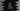# C++ program to print 1 to 100 in different ways## C++ program to print 1 to 100:

In this post, we will learn how to print from 1 to 100 in C++. We will learn different ways to solve this problem.

Let’s try to print 1 to 100 by using a for loop. The for loop checks one condition, if the condition is true, it runs the code in its body.

Normally, we use a variable with an initial value in a for loop. On each iteration of the loop, this value is changed. The loop stops if this value reaches at some predefined value.

For example, we can run one for loop from i = 0 to i = 100, incrementing the value of i by 1 in each iteration.

for loop is defined as like below:

``````for(initialization, loop exit condition, run after each iteration ){
// body
}``````
• initialization is the part to initialize a variable or any other condition.
• loop exit condition is a condition, the loop will stop if it is false.
• run after each iteration part is to run after each iteration, i.e. we can increment/decrement a variable

Below is the complete program:

``````#include <iostream>

using namespace std;

int main()
{
for (int i = 1; i <= 100; i++)
{
cout << i << endl;
}
}``````

Here,

• We are running a for loop from i = 1 to i <=100.
• The value of i is initialized as 1. It will run until the value of i is less than or equal to 100. After each iteration, it is incrementing the value of i by 1.
• In the body part, it is printing the value of i using cout. Since, the loop runs from i = 1 to i = 100, printing the value of i will print all numbers from 1 to 100.
• The endl will add one new line with the printed value.

If you run this program, it will print:

``````1
2
3
4
5
6
7
..
..``````

It will print all from 1 to 100.

We can also print 1 to 100 by using a while loop.

while loop also works based on a condition. It checks one condition and if that condition is true, it runs the body of the loop. If it is false, it stops the execution.

It will work similarly to the for loop. The initialization is done before the loop starts and at the end of the while loop, it will increment the variable by 1.

It is defined as:

``````while(condition){
//body
}``````

Let’s write down the program using a while loop:

``````#include <iostream>

using namespace std;

int main()
{
int i = 1;
while (i <= 100)
{
cout << i << endl;
i++;
}
}``````

It will print similar output.

If you look closely, both for loop and while loop works in a similar way.

do-while loop is similar to while loop. It is defined as like below:

``````do{
//body
}while(condition)``````

It keeps running the body of the loop until the condition returns true. In a while loop, it checks the condition first and runs the body of the loop, but in a do-while, it runs the body and then checks for the condition.

We can print from 1 to 100 by using a do-while loop:

``````#include <iostream>

using namespace std;

int main()
{
int i = 1;
do
{
cout << i << endl;
i++;
} while (i <= 100);
}``````